## Preparations and initialisation

In order to be able to execute the following code, one first needs to install the following packages (along with betaboost):

## Install packages
install.packages(c("betareg"))       ## install betareg for comparison of results


Next, we load the betaboost package

library("betaboost")                 ## load betaboost


## Example 1: Comparison of various boosted models

First, we analyse publicly available data on health-realated quality of life of 60 patients. The data set was originally published as dataqol2 in the QoLR package and later added to betareg. The explanatory variables are the treatment group (arm) and an additional score measuring the patients pain. First, we load and preprocess the data:

## load data
# data("dataqol2", package = "QoLR")
data(dataqol2)
## take one time-point
dataqol <- dataqol2[dataqol2$time ==0,] ## remove missings dataqol <- dataqol[complete.cases(dataqol[,c("QoL", "arm", "pain")]),] ## rescale outcome to [0,1] dataqol$QoL <- dataqol$QoL/100  ### Raw data The data set consists of 7 variables and 57 observations. str(dataqol)  ## 'data.frame': 57 obs. of 7 variables: ##$ id   : int  1 2 4 5 7 8 9 10 11 12 ...
##  $time : int 0 0 0 0 0 0 0 0 0 0 ... ##$ date : int  0 0 0 0 0 0 0 0 0 0 ...
##  $QoL : num 0.78 0.67 0.6 0.59 0.51 0.51 0.53 0.59 0.87 0.48 ... ##$ pain : int  36 36 34 36 27 31 18 50 31 23 ...
##  $arm : int 0 0 0 0 0 0 0 0 0 0 ... ##$ death: int  251 NA NA NA NA 123 NA NA 240 NA ...


The raw outcome is distributed as follows (histogram of the Quality of Life with smooth kernel density estimate for its empirical distribution).

hist(dataqol$QoL, breaks = 20, col = "lightgrey", main = "Quality of life", prob = TRUE, las = 1) lines(density(dataqol$QoL), col = 2, lwd = 2)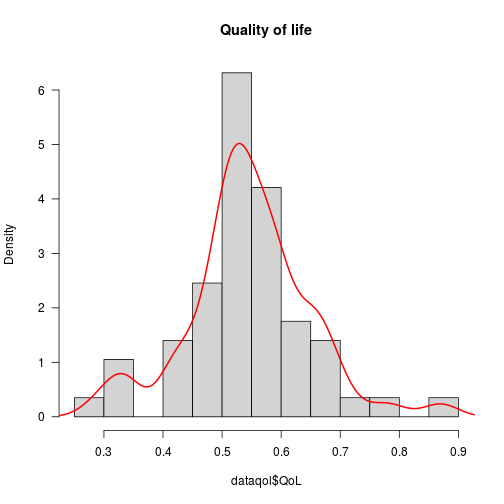The influence of various predictors on the outcome is depicted in the following: First, boxplots comparing the Quality of Life outcome for both treatment arms.

## QOL by treatment arm
boxplot(QoL ~ arm, data = dataqol, names = c("A","B"), las = 1,
col = "lightgrey", ylab = "Qualitiy of life")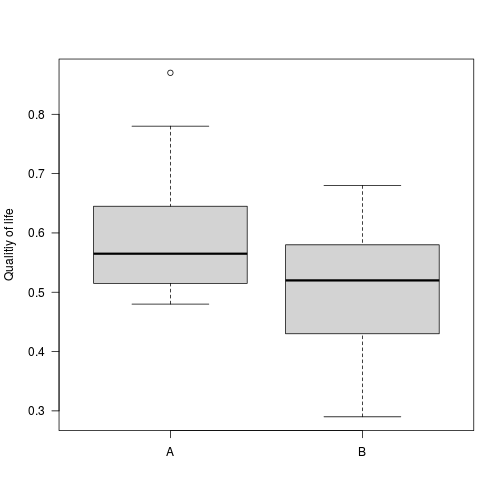Second, a scatterplot for the relationship between the explanatory variable pain (x-axis) and the outcome variable (y-axis):

## QOL by pain
plot(QoL ~ pain, data = dataqol, pch = 19, las = 1)
abline(lm(QoL ~ pain, data = dataqol))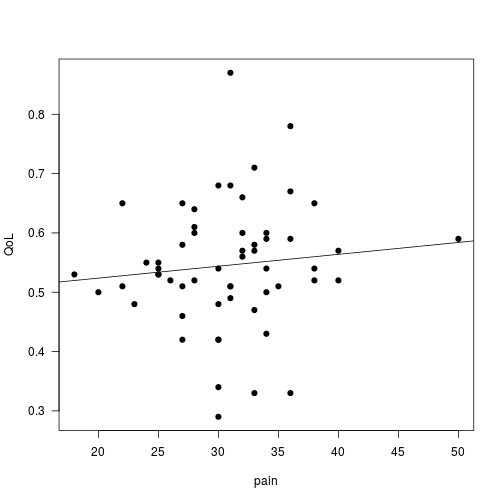### Boosted beta models

First, we fit a classical beta regression with linear effects

beta1 <- betaboost(QoL ~ pain + arm, data = dataqol)
coef(beta1, off2int = TRUE)

## (Intercept)        pain         arm
##  0.19037893  0.00529943 -0.33447873


the precission parameter is treated as nuissance.

nuisance(beta1)

##  24.73453


Note, that here we automatically are using 100 boosting iterations mstop = 100, as this is the default value.

Similarly, we can fit a model with smooth effect for pain

beta2 <- betaboost(QoL ~ s(pain) + arm, data = dataqol,
form.type = "classic")


and plot the corresponding smooth effect for the explanatory variable pain on the outcome QoL.

plot(beta2, which = "pain")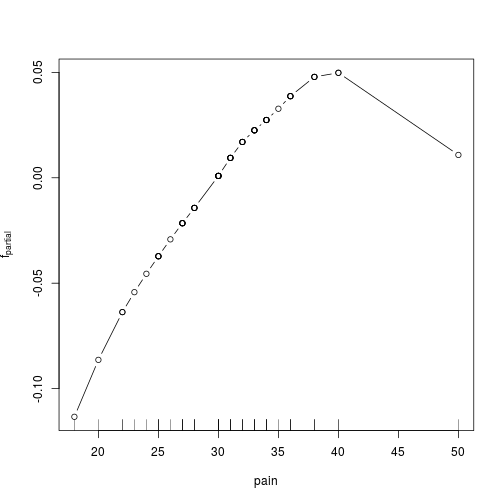The effect represents the estimate f(pain) evaluated at the different observations. This effect does not vary with other variables, but depends only on the pain value.

As for all boosting methods, we need to tune the model regarding the number of boosting iterations mstop via cross-validation or bootstrap procuedures which are implemented in cvrisk(). Note that the function uses 25-fold bootstrap per default.

This takes a few seconds.

set.seed(1234)
cvr <- cvrisk(beta2)
## extract optimal boosting iteration as determined via cvrisk
mstop(cvr)

##  10

## and plot the predictive risk (on out-of-bag observations) over the iterations
plot(cvr)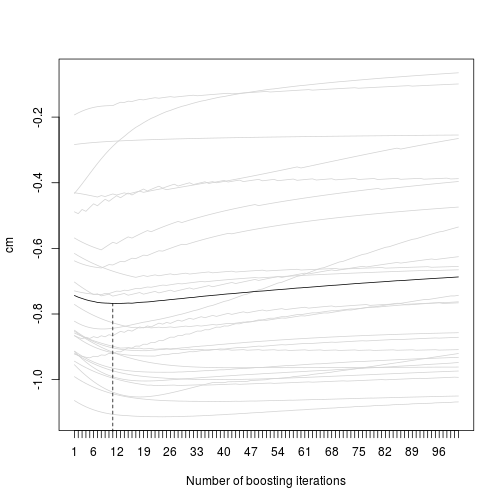The plot displays results from 25-fold bootstrap to optimize the number of boosting iterations. The gray lines indicate the performance (regarding predictive risk) for the 25 bootstrap samples. The solid black line represents the average performance; the optimal performance (minimal risk) is reached after 10 iterations (dashed vertical line).

Afterwards, we set the model to the optimal iteration:

## set model to optimal stopping iteration:
mstop(beta2) <- mstop(cvr)


As a result of early stopping, variable selection takes place and pain is no longer included in the final model:

coef(beta2)

## $bols(arm) ## (Intercept) arm ## 0.0788887 -0.1524986 ## ## attr(,"offset") ##  0.1822572  Now, we fit an extended beta regression model, where the precision parameter phi is modeled as well. This is achieved by additionally providing a phi.formula: beta3 <- betaboost(QoL ~ arm + pain, phi.formula = QoL ~ arm + pain, data = dataqol) coef(beta3, off2int = TRUE)  ##$mu
##  (Intercept)          arm         pain
##  0.194675488 -0.329714708  0.005154894
##
##        [,1]
## 1 0.5864532
## 2 0.5049017
##
## $phi ## [,1] ## 1 22.00234 ## 2 22.00234  As arm was not selected for the precision part of the model, only mu is influenced by the treatment arm, phi stays the same. ### Compare R$$^2$$ measures for the four boosting models cbind("lin" = R2.betaboost(beta1, data = dataqol), "smooth" = R2.betaboost(beta2, data = dataqol), "ext. lin" = R2.betaboost(beta3, data = dataqol), "ext. smooth" = R2.betaboost(beta4, data = dataqol))  ## lin smooth ext. lin ext. smooth ## R2_maddala 0.1635197 0.1661942 0.1502778 0.150995 ## R2_link 0.1594109 0.162418 0.1592298 0.1620651 ## R2_response 0.1633067 0.1653875 0.1632926 0.1654617  ## Example 2: Comparison of betareg and betaboost The second data set is a standard example for beta regression. The outcome is the proportion of income spent on food for a random sample of 38 households in a large US city. The data set is freely available and included in the betareg package. ## load betareg and data library(betareg) data(FoodExpenditure)  First, we fit the standard beta regression model to the data with betareg: beta1 <- betareg(I(food/income) ~ income + persons, data = FoodExpenditure)  Now, we fit a boosted beta regression model with betaboost: beta2 <- betaboost(I(food/income) ~ income + persons, data = FoodExpenditure)  If we compare the estimated coefficients of the methods rbind("betareg" = coef(beta1), "betaboost" = c(coef(beta2, off2int = TRUE), nuisance(beta2)))  ## (Intercept) income persons (phi) ## betareg -0.6225481 -0.01229884 0.1184621 35.60975 ## betaboost -0.6203121 -0.01167270 0.1113287 35.23329  we see that there are only minor differences. If we increase the number of boosting iterations to 500 iterations, the boosting model converges to the standard solution of betareg: mstop(beta2) <- 500 ## compare again rbind("betareg" = coef(beta1), "betaboost" = c(coef(beta2, off2int = TRUE), nuisance(beta2)))  ## (Intercept) income persons (phi) ## betareg -0.6225481 -0.01229884 0.1184621 35.60975 ## betaboost -0.6225479 -0.01229870 0.1184606 35.60968  The following graphic is a coefficient plot for 500 boosting iterations (coefficient estimates on the y-axis and number of boosting iteration on the x axis). One can observe how the algorithm rapidly converges to the same effect estimates as those obtained from the standard software betareg. In fact, after the first 100 boosting iterations there are only minor changes in the coefficients : plot(beta2, off2int = TRUE, main = "boosting")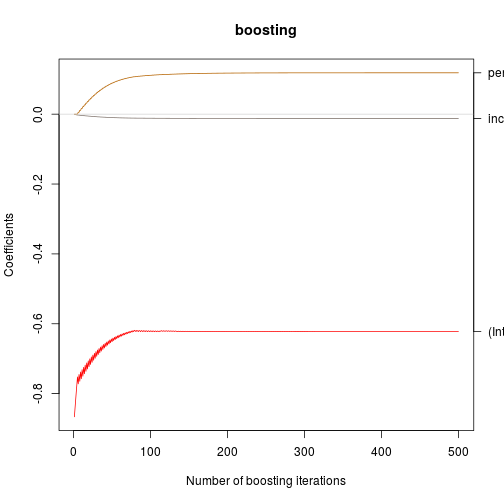Note that betaboost incorporates additionally also a matrix interface, which is particularly advantageous in case of larger or even high-dimensional models. Instead of using the formula interface, the user only needs to provide the response y and a matrix x. Via mat.parameter = c("mean","both") and mat.effect = c("linear","smooth") the user can specify weather to apply classical or extended beta regression, and if linear or non-linear effects are assumed. ## Fit same model than beta2 but via matrix interface beta2b <- betaboost(y = FoodExpenditure$food/FoodExpenditure$income, x = cbind(FoodExpenditure$income, FoodExpenditure$persons), iterations = 500) coef(beta2b)  ## intercept ## 0.2744383 -0.0122987 0.1184606 ## attr(,"offset") ##  -0.8969861  ## Now extended beta regression beta2c <- betaboost(y = FoodExpenditure$food/FoodExpenditure$income, x = cbind(income = FoodExpenditure$income, persons = FoodExpenditure$persons), iterations = 500, mat.parameter = "both", mat.effect = "linear") coef(beta2c)  ##$mu
## (Intercept)      income     persons
##  0.16840877 -0.01004507  0.11071215
## attr(,"offset")
##  -0.8876991
##
## \$phi
## (Intercept)     persons
##   1.1508957  -0.2053655
## attr(,"offset")
##  3.03731